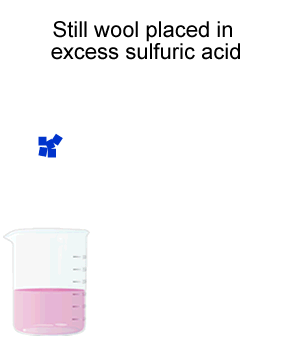Volumetric analysis

Backtitration

This technique involves the deliberate addition of excess reagent to the test sample. After the reaction is complete the amount in excess is then determined by titration.

A feature of this technique is that we use excess reagent and two stoichiometric equations.We generally use this technique when we are dealing with very weak acids and bases that are unable to make an indicator change colour sharply at the end point of a titration.
Lets see an example, although in this example we are using a strong acid it is just the process that we are concerned about.

A 20.0g piece of steel wool is dissolved in 300.0mL of 1.00M sulphuric acid, H2SO4. The excess sulphuric acid is determined by titration with a 0.500M NaOH solution, as shown on the right. 280.0mL of sodium hydroxide is required to neutralise the acid. What was the percentage, by mass, of iron in the steel wool?

Step 1) Find the mole of NaOH used to neutralise the excess acid.
(n = c v)  0.500 x 0.280 = 0.140

Step 2 Derive the equation of the reaction between NaOH and H2SO4.

2NaOH(aq) + H2SO4(aq)  => Na2SO4(aq) + 2H2O(l)

Step 3 From the equation above find the excess H2SO4.
(n=cv)  ½ x 0.140 = 0.0700

Step 4 Calculate the initial amount of H2SO4 .used
(n=cv)  1.00 x 0.300 = 0.300

Step 5 Calculate the amount of  H2SO4 that reacted.
0.300 – 0.0700 = 0.230

Step 6 Derive the equation for the reaction between H2SO4 and Fe

Fe(s) + H2SO4.(aq)  => FeSO4(aq) + H2(g)

Step 7 Calculate the mol of Fe in steel wool  1/1 x 0.230 =0.230
Step 8 Calculate the mass of Fe (m=n x Fm)  0.230 x 55.8 = 12.83g
Step 9 Calculate the % of Fe   (12.83 / 20.0) x 100/1 = 64.2%3.00g sample of impure limestone is treated with 100ml of 1.1M HCl solution. The reaction takes place according to the equation below.

CaCO3(aq) + 2HCl(aq) => CaCl2(aq) + H2O(l) + CO2(g)

After the reaction is complete it is found that 20.10mls of a 1.50M sodium carbonate solution is required to neutralise the unreacted acid.

Calculate the percentage of calcium carbonate in the sample.

(Ca = 40.0, C= 12.0, O=16.0, CL =35.5, H =1.0)

 Volume Start 12.02mls Finish 32.12 mls Total 20.10mls

Home